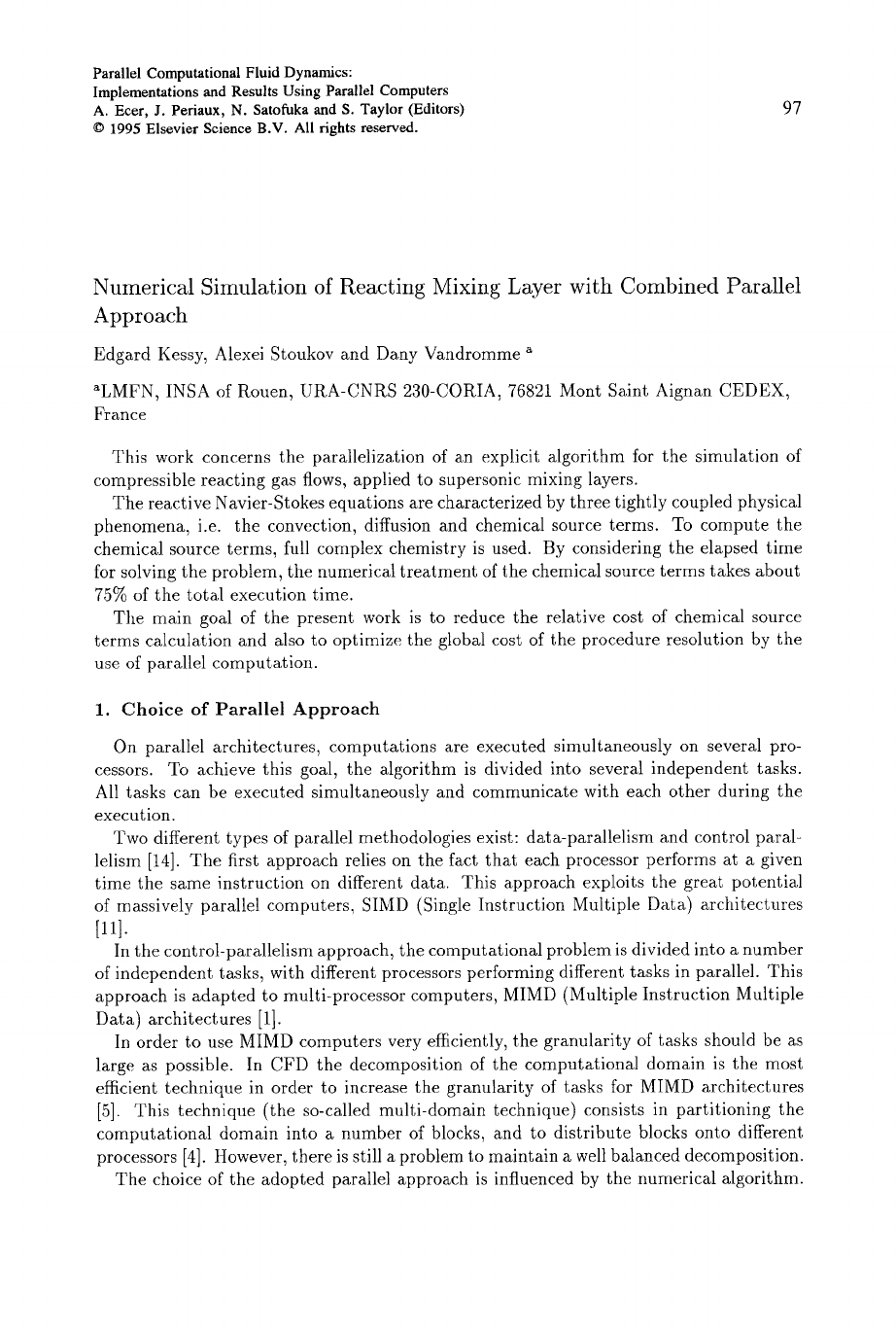Parallel Computational Fluid Dynamics:
Implementations and Results Using Parallel Computers
A. Ecer, J. Periaux, N. Satofuka and S. Taylor (Editors)
97
Numerical Simulation of Reacting Mixing Layer with Combined Parallel
Approach
Edgard Kessy, Alexei Stoukov and Dany Vandromme ~
~LMFN, INSA of Rouen, URA-CNRS 230-CORIA, 76821 Mont Saint Aignan CEDEX,
France
This work concerns the parallelization of an explicit algorithm for the simulation of
compressible reacting gas flows, applied to supersonic mixing layers.
The reactive Navier-Stokes equations are characterized by three tightly coupled physical
phenomena, i.e. the convection, diffusion and chemical source terms. To compute the
chemical source terms, full complex chemistry is used. By considering the elapsed time
for solving the problem, the numerical treatment of the chemical source terms takes about
75% of the total execution time.
The main goal of the present work is to reduce the relative cost of chemical source
terms calculation and also to optimize the global cost of the procedure resolution by the
use of parallel computation.
1. Choice of Parallel Approach
On parallel architectures, computations are executed simultaneously on several pro-
cessors. To achieve this goal, the algorithm is divided into several independent tasks.
All tasks can be executed simultaneously and communicate with each other during the
execution.
Two different types of parallel methodologies exist: data-parallelism and control paral-
lelism . The first approach relies on the fact that each processor performs at a given
time the same instruction on different data. This approach exploits the great potential
of massively parallel computers, SIMD (Single Instruction Multiple Data) architectures
.
In the control-parallelism approach, the computational problem is divided into a number
of independent tasks, with different processors performing different tasks in parallel. This
approach is adapted to multi-processor computers, MIMD (Multiple Instruction Multiple
Data) architectures .
In order to use MIMD computers very efficiently, the granularity of tasks should be as
large as possible. In CFD the decomposition of the computational domain is the most
efficient technique in order to increase the granularity of tasks for MIMD architectures
. This technique (the so-called multi-domain technique) consists in partitioning the
computational domain into a number of blocks, and to distribute blocks onto different
processors . However, there is still a problem to maintain a well balanced decomposition.
The choice of the adopted parallel approach is influenced by the numerical algorithm.98
However, one can notice that data-parallel and control-parallel approaches can both be
combined . By considering that in most reacting flows, reacting and non-reacting zones
occur simultaneously, the computation of chemical source terms can be restricted to the
reactive region. Thus a decomposition efficient for pure hydro dynamical problem becomes
inefficient when the reacting zone dimensions differ greatly between blocks. In such a way,
the standard multi-block technique is no longer well suited for the reacting flow. In this
paper, a multi-block technique is used for convective and diffusive terms, whereas SPMD
(Single Program Multiple Data) or SIMD approach is employed for chemical source terms.
Figure 1. Flow configuration
2. Flow configuration, physical, chemical and mathematical model
Figure 1 shows the physical model considered in the present study. It consists of two
chemically active hydrogen and air streams with different stream wise velocities. The
spatial mixing of reacting streams has been simulated in a two-dimensional domain.
The static pressure at the inlet side is the same
for both streams. To prescribe the inlet condi-
tions the self similar solution of the compress-
ible mixing layer  is used at the inlet with
a vorticity thickness equal to 0.05 of the trans-
verse length. The inlet conditions are presented
in Table 1.
Table 1
Stream Velocity Mach Pressure Temperature Convective
m/s
number
Pa K
Mach number
/-/2 3000 1.24 1.013-l0 s 930- 1000 0.446
AIR
1780 2.803
The flow evolution is governed by the unsteady compressible Navier-Stokes equations
coupled with the energy and species transport equations. These equations are written in
two-dimensional form as:
OU OF(U) OG(U) = S(U)
(1)
O----t -~ Ox t Oy
where the variable vector, the convective and diffusive fluxes and source terms are defined
respectively by:
U = [Pl,""",
Pnsp, pu, pv, pet] T
F=Fc+Fv G=Gc+Gv
Fc
= [plu,"',
PnspU, pUU + P, puv, (pet + p)u] T
[
Fv = - ~rylx, . . . , ~ryn~.px , ~rx~, cr~y, cr~u + axvv - q~
Gc
= [Plv,"',
PnspV, pVU, pVV -t- p, (pet + p)v] T

Get Parallel Computational Fluid Dynamics '95 now with the O’Reilly learning platform.

O’Reilly members experience books, live events, courses curated by job role, and more from O’Reilly and nearly 200 top publishers.